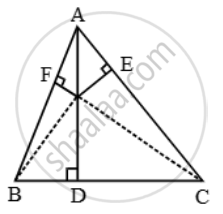From a point O in the interior of a ∆ABC, perpendicular OD, OE and OF are drawn to the sides BC, CA and AB respectively - Mathematics

Sum

From a point O in the interior of a ∆ABC, perpendicular OD, OE and OF are drawn to the sides BC, CA and AB respectively. Prove
that :

(i) AF^2 + BD^2 + CE^2 = OA^2 + OB^2 + OC^2 – OD^2 – OE^2 – OF^2

(ii) AF^2 + BD^2 + CE^2 = AE^2 + CD^2 + BF^2

SolutionLet O be a point in the interior of ∆ABC and let OD ⊥ BC, OE ⊥ CA and OF ⊥ AB.

(i) In right triangles ∆OFA, ∆ODB and ∆OEC, we have

OA^2 = AF^2 + OF^2

OB^2 = BD^2 + OD^2

and,

OC^2 = CE^2 + OE^2

Adding all these results, we get

OA^2 + OB^2 + OC^2 = AF^2 + BD^2 + CE^2 + OF^2 + OD^2 + OE^2

⇒ AF^2 + BD^2 + CE^2 = OA^2 + OB^2 + OC^2 – OD^2 – OE^2 – OF^2

(ii) In right triangles ∆ODB and ∆ODC, we have

OB^2 = OD^2 + BD^2

and, OC^2 = OD^2 + CD^2

OB^2 – OC^2 = (OD^2 + BD^2 ) – (OD^2 + CD^2 )

⇒ OB^2 – OC^2 = BD^2 – CD^2 ….(i)

Similarly, we have

OC^2 – OA^2 = CE^2 – AE^2 ….(ii)

and, OA^2 – OB^2 = AF^2 – BF^2 ….(iii)

Adding (i), (ii) and (iii), we get

(OB^2 – OC^2 ) + (OC^2 – OA^2 ) + (OA^2 – OB^2 )

= (BD^2 – CD^2 ) + (CE^2 – AE^2 ) + (AF^2 – BF^2 )

⇒ (BD^2 + CE^2 + AF^2 ) – (AE^2 + CD^2 + BF^2 ) = 0

⇒ AF^2 + BD^2 + CE^2 = AE^2 + CD^2 + BF^2

Concept: Right-angled Triangles and Pythagoras Property
Is there an error in this question or solution?